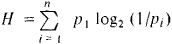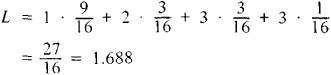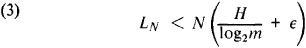coding

(redirected from codings)
Also found in: Dictionary, Thesaurus, Medical.

coding

[′kōd·iŋ]
(computer science)
The process of converting a program design into an accurate, detailed representation of that program in some suitable language.
A list, in computer code, of the successive operations required to carry out a given routine or solve a given problem.
McGraw-Hill Dictionary of Scientific & Technical Terms, 6E, Copyright © 2003 by The McGraw-Hill Companies, Inc.

coding

the assignment of (generally) numerical codes to specific data in such a way as to allow analysis to be undertaken by means of computer or by hand. The need to code data in a meaningful way is common to much sociological research, whether it is describing a phenomenon or testing a sociological theory.

It is possible to differentiate between two basic types of coding – structured and unstructured – depending upon the type of data which is to be analysed, although the difference between them is blurred. STRUCTURED CODING can generally be used on primary data, i.e. that which the researcher collects directly Unstructured coding is generally used with data which has been collected by the researcher from secondary sources. The main example of the use of structured coding is in QUESTIONNAIRE analysis, and that of unstructured coding in CONTENT ANALYSIS. See also UNSTRUCTURED DATA.

Collins Dictionary of Sociology, 3rd ed. © HarperCollins Publishers 2000
The following article is from The Great Soviet Encyclopedia (1979). It might be outdated or ideologically biased.

Coding

(encoding), the operation of identifying the symbols or groups of one code with the symbols or groups of another code. The need for coding arises primarily because the form of a message must be adapted to a particular communications channel or to some other facility designed for the transformation or storage of information. Thus, messages presented in the form of a series of letters (such as Russian) and numbers are transformed by means of telegraph codes into certain combinations of current pulses. Numerical data that are entered in computers are usually transformed from the decimal system into the binary system.

Coding is used in information theory to reduce the redundancy of messages and the effect of noise, which distorts messages during transmission over communications channels. Consequently, the selection of a new code is an attempt to match it more successfully with the statistical structure of the message source in question. To a certain extent this matching has already been done in telegraphic code, where the most common letters are designated by the shortest combinations of dots and dashes.

The methods used in information theory to produce the matching mentioned above can be illustrated by an example of the construction of “economical” binary codes. Let us assume that a channel can transmit only the symbols 0 and 1, expending the same time t on each. To reduce the transmission time (or to increase the rate of transmission, which is equivalent), it is advantageous to code the messages before transmission in such a manner that the average length L of the code notation is at a minimum. Let x1, x2, . . . ,xn designate the possible messages from a certain source and p1, p2, . . . ,pn indicate their corresponding probabilities. Then, as established by information theory, for any method of coding,

(1) L ≥ H

whereis the entropy of the source. The boundary for L in equation (1) may not be reached. However, for any pi a method of coding exists (the Shannon-Fano method) such that

(2) L ≤ H+1

In this method, the messages are arranged in order of decreasing probability and the series thus obtained is divided into two parts whose probabilities are as close as possible to one another. The first binary symbol is taken as 0 in the first part and 1 in the second part. In a similar way each of the parts is divided in half and a second binary symbol is chosen, and so on, until parts containing only one message are reached.

Example 1. Let n = 4 and p1 = 9/16, p2 = p3 = 3/16, and p4 = 1/16. The application of the method is illustrated in Table 1.

Table 1
xipi Code notation
x1 . . . . . . . . . . . . . . . . . . . .9/160
x2 . . . . . . . . . . . . . . . . . . . .3/1610
x3 . . . . . . . . . . . . . . . . . . . .3/16110
x4 . . . . . . . . . . . . . . . . . . . .1/16111

In this caseand it can be shown that no other code will give a smaller value. Here H = 1.623. All of the above applies to a case in which the alphabet of a new code contains not two letters, as assumed above, but m > 2 letters. In this case only the quantity H in equations (1) and (2) should be replaced by the quantity H/log 2m.

The problem of “contraction” of the notation of messages in a given alphabet (the problem of reducing redundancy) can be solved on the basis of the Shannon-Fano method. Indeed, if messages are represented by a series of letters of length N from an m-letter alphabet, then their average length LN after coding always satisfies the inequality LNNH/log2m where H is the entropy of the source per letter. On the other hand, if ∊ > 0 as closely as desired, all values of N that are large enough may satisfy the inequalityCoding by “blocks,” in which, depending on ∊, a positive integer s is chosen and every message is divided into an equal number of parts, or “blocks,” containing s letters, is used for this purpose. These blocks are then coded by the Shannon-Fano method into the same alphabet. For sufficiently large N, inequality (3) will be satisfied. The validity of this assertion is most easily understood by considering a case in which the source is a sequence of independent symbols 0 and 1, which appear with probabilities p and q, respectively, where pq. The entropy per block is equal to the entropy for one letter multiplied by s —that is, sH = s(p log2 1/P + q log21/q). The code notation of a block requires, on the average, no more than sH + 1 binary symbols. Therefore, for a message of length N letters, LN ≤ (1 + N/s)(sH + 1) = N(H + 1/s)(1 + s/N), which for sufficiently large s and N/s leads to the inequality of (3). For such a code the entropy per letter approaches its maximum value, unity, and the redundancy approaches zero.

Example 2. Let the message source be a sequence of independent symbols 0 and 1 in which the probability of the appearance of 0 is p = ¾ and for 1 it is q = ¼. Here the entropy per letter H is equal to 0.811, and the redundancy is 0.189. The smallest blocks (s = 2)—that is, 00, 01, 10, and 11—have probabilities p2 = 9/16, pq = 3/16, qp = 3/16, and q2 = 1/16, respectively. The application of the Shannon-Fano method (see Example 1) leads to the coding rule 00 → 0, 01 → 10, 10 → 110, and 11 → 111. In this case, for example, the message 00111000 . . . assumes the form 01111100 . . . . For each letter of the message in the previous form there is on the average 27/32 = 0.844 letter in the new form (with a lower limit for the contraction coefficient H — 0.811). The entropy per letter in the new sequence is 0.811/0.844 = 0.961, and the redundancy is 0.039.

Coding that reduces interference has become a large section of information theory, with its own mathematical apparatus, which to a considerable extent is purely algebraic.

IU. V. PROKHOROV